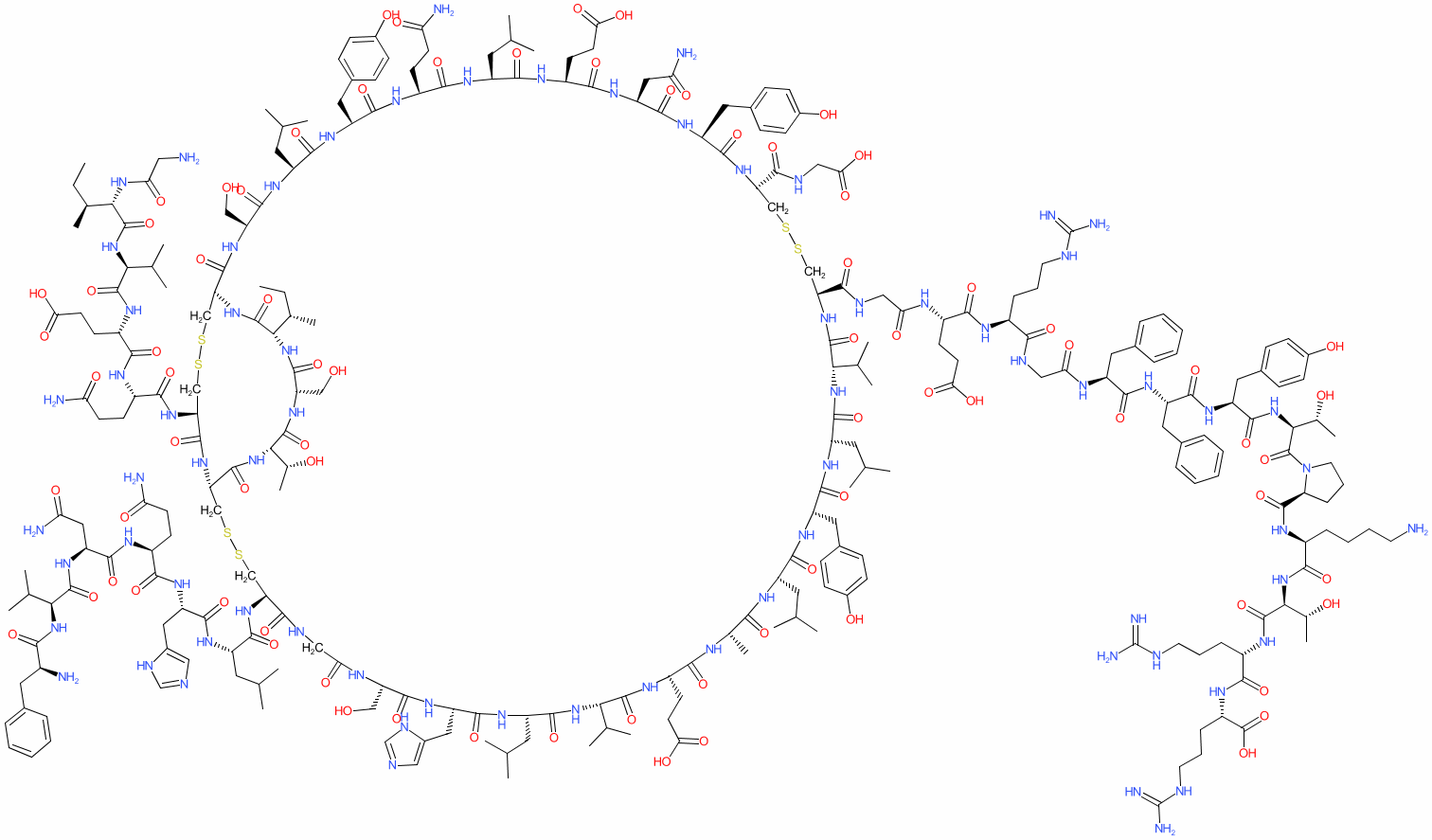#### GtoPdb Ligand ID: 7572

Synonyms: HOE-901 | HOE901 | Lantus®
insulin glargine is an approved drug (FDA and EMA (2000))
Comment: Insulin glargine is a recombinant, modified analogue of human insulin. The chemical structure shown here was generated using the SMILES from PubChem CID 44146714.

A number of insulin glargine biosimilars have been approved internationally since the expiration of patents on Lantus® in 2014: a comprehensive list that is maintained by the Generics and Biosimilar Initiative (https://www.gabionline.net) is available here. The first fully interchangeable biosimilar to be FDA approved was Semglee® (insulin glargine-yfgn; Mylan Pharmaceuticals), in July 2021.View more information in the IUPHAR Pharmacology Education Project: glargine

2D StructureSMILES / InChI / InChIKeyCanonical SMILES NCCCC[C@@H](C(=O)N[C@H](C(=O)N[C@H](C(=O)N[C@H](C(=O)O)CCCNC(=N)N)CCCNC(=N)N)[C@H](O)C)NC(=O)[C@@H]1CCCN1C(=O)[C@H]([C@H](O)C)NC(=O)[C@@H](NC(=O)[C@@H](NC(=O)[C@H](Cc1ccccc1)NC(=O)CNC(=O)[C@@H](NC(=O)[C@@H](NC(=O)CNC(=O)[C@@H]1CSSC[C@H](NC(=O)[C@H](Cc2ccc(cc2)O)NC(=O)[C@H](CC(=O)N)NC(=O)[C@H](CCC(=O)O)NC(=O)[C@H](CC(C)C)NC(=O)[C@H](CCC(=O)N)NC(=O)[C@H](Cc2ccc(cc2)O)NC(=O)[C@H](CC(C)C)NC(=O)[C@H](CO)NC(=O)[C@@H]2CSSC[C@H](NC(=O)[C@@H](NC(=O)[C@@H](NC(=O)[C@H](C(C)C)NC(=O)[C@H]([C@H](CC)C)NC(=O)CN)CCC(=O)O)CCC(=O)N)C(=O)N[C@@H](CSSC[C@@H](C(=O)NCC(=O)N[C@H](C(=O)N[C@H](C(=O)N[C@H](C(=O)N[C@H](C(=O)N[C@H](C(=O)N[C@H](C(=O)N[C@H](C(=O)N[C@H](C(=O)N[C@H](C(=O)N[C@H](C(=O)N1)C(C)C)CC(C)C)Cc1ccc(cc1)O)CC(C)C)C)CCC(=O)O)C(C)C)CC(C)C)Cc1[nH]cnc1)CO)NC(=O)[C@@H](NC(=O)[C@@H](NC(=O)[C@@H](NC(=O)[C@@H](NC(=O)[C@H](C(C)C)NC(=O)[C@H](Cc1ccccc1)N)CC(=O)N)CCC(=O)N)Cc1[nH]cnc1)CC(C)C)C(=O)N[C@H](C(=O)N[C@H](C(=O)N[C@H](C(=O)N2)[C@H](CC)C)CO)[C@H](O)C)C(=O)NCC(=O)O)CCC(=O)O)CCCNC(=N)N)Cc1ccccc1)Cc1ccc(cc1)O Isomeric SMILES CC[C@H](C)[C@H]1C(=O)N[C@H]2CSSC[C@@H](C(=O)N[C@@H](CSSC[C@@H](C(=O)NCC(=O)N[C@H](C(=O)N[C@H](C(=O)N[C@H](C(=O)N[C@H](C(=O)N[C@H](C(=O)N[C@H](C(=O)N[C@H](C(=O)N[C@H](C(=O)N[C@H](C(=O)N[C@H](C(=O)N[C@@H](CSSC[C@H](NC(=O)[C@@H](NC(=O)[C@@H](NC(=O)[C@@H](NC(=O)[C@@H](NC(=O)[C@@H](NC(=O)[C@@H](NC(=O)[C@@H](NC(=O)[C@@H](NC2=O)CO)CC(C)C)Cc2ccc(cc2)O)CCC(=O)N)CC(C)C)CCC(=O)O)CC(=O)N)Cc2ccc(cc2)O)C(=O)NCC(=O)O)C(=O)NCC(=O)N[C@@H](CCC(=O)O)C(=O)N[C@@H](CCCNC(=N)N)C(=O)NCC(=O)N[C@@H](Cc2ccccc2)C(=O)N[C@@H](Cc2ccccc2)C(=O)N[C@@H](Cc2ccc(cc2)O)C(=O)N[C@@H]([C@@H](C)O)C(=O)N2CCC[C@H]2C(=O)N[C@@H](CCCCN)C(=O)N[C@@H]([C@@H](C)O)C(=O)N[C@@H](CCCNC(=N)N)C(=O)N[C@@H](CCCNC(=N)N)C(=O)O)C(C)C)CC(C)C)Cc2ccc(cc2)O)CC(C)C)C)CCC(=O)O)C(C)C)CC(C)C)Cc2cnc[nH]2)CO)NC(=O)[C@H](CC(C)C)NC(=O)[C@H](Cc2cnc[nH]2)NC(=O)[C@H](CCC(=O)N)NC(=O)[C@H](CC(=O)N)NC(=O)[C@H](C(C)C)NC(=O)[C@H](Cc2ccccc2)N)C(=O)N[C@H](C(=O)N[C@H](C(=O)N1)CO)[C@@H](C)O)NC(=O)[C@H](CCC(=O)N)NC(=O)[C@H](CCC(=O)O)NC(=O)[C@H](C(C)C)NC(=O)[C@H]([C@@H](C)CC)NC(=O)CN InChI Key COCFEDIXXNGUNL-RFKWWTKHSA-N
 ClassificationCompound class Peptide or derivative Approved drug? Yes (FDA and EMA (2000))
 International Nonproprietary NamesINN number INN 7548 insulin glargine
 SynonymsHOE-901 | HOE901 | Lantus®
 Database LinksCAS Registry No. 160337-95-1 ChEMBL Ligand CHEMBL1201497 DrugBank Ligand DB00047 GtoPdb PubChem SID 223365908 PubChem CID 44146714 Search Google for chemical match using the InChIKey COCFEDIXXNGUNL-RFKWWTKHSA-N Search Google for chemicals with the same backbone COCFEDIXXNGUNL Search PubMed clinical trials insulin glargine Search PubMed titles insulin glargine Search PubMed titles/abstracts insulin glargine UniChem Compound Search for chemical match using the InChIKey COCFEDIXXNGUNL-RFKWWTKHSA-N UniChem Connectivity Search for chemical match using the InChIKey COCFEDIXXNGUNL-RFKWWTKHSA-N Wikipedia Insulin glargine